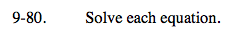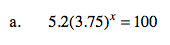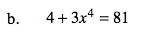Home > A2C > Chapter 9 > Lesson 9.2.1 > Problem9-80

9-80.
1. Solve each equation. Homework Help ✎

1. 5.2(3.75)x = 100

2. 4 + 3x4 = 81Divide by 5.2 and then use logarithms.

x ≈ 2.24Use roots or fractional exponents.

$\textit{x}=\pm\sqrt[\text{4}]{\frac{77}{3}}\approx\pm2.25$